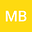Equivalences and differences between the hydrological dynamical systems of water budget, travel time, response time, and tracer concentrations and the legacy of models' topology
••Riccardo RigonUniversità di Trento, Dipartimento di Ingegneria Civile, Ambientale e Meccanica, University of Trento

Corresponding Author:[email protected]

Author Profile## Abstract

We present, by using previous results on extended Petri Nets, the relations of various hydrological dynamical systems ($\mathtt{HDSys}$) derived from the water budget ($\mathtt{DynWB}$). Once $\mathtt{DynWB}$ has been implemented, there exist a consistent way to get the equations for backward travel time distributions ($\mathtt{DynTT}$), for the forward response time distribution ($\mathtt{DynRTD}$) and for the concentration for a solute or a tracer ($\mathtt{DynC}$). We show that the $\mathtt{DynWB}$ has a correspondence one to many with the $\mathtt{DynTT}$. In fact to any one of the $\mathtt{DynWB}$ equation correspond as many equation as the input precipitation events times. The $\mathtt{DynTT}$ is related to $\mathtt{DynRTD}$ by the Niemi’s relationship and, in presence of multiple, $n$ outputs, by the specification of $n-1$ partition functions, which determine which fraction of water volume, injected in the control volume at a specific time $t_{in}$, goes asymptotically into a specific output. The $\mathtt{DynC}$, given $\mathtt{DynTT}$, depends further on the solute/tracer concentration in inputs. The paper clarifies the complicate set of relations above by using an example from literature. Upon the introduction of the appropriate information, it is also shown how these ($\mathtt{HDSys}$) can be solved simultaneously without duplicating calculations. It is also shown that these systems can be solved exactly, under the hypothesis of uniform mixing of water ages inside each reservoir within the system.
12 May 2020Submitted to Hydrological Processes
14 May 2020Submission Checks Completed
14 May 2020Assigned to Editor
14 May 2020Reviewer(s) Assigned
14 Aug 2020Review(s) Completed, Editorial Evaluation Pending
27 Aug 2020Editorial Decision: Revise Major